## Pages

### Factoring Trinomials of the Form x^2 + bx + c

In this section we will factor trinomials - polynomials with three terms. Students find this difficult at first. However, with much practice factoring trinomials becomes routine. If a trinomial factors, then it will factor into the product of two binomials.

Factor:Step 1: Factor the first term: x^2 = x*x.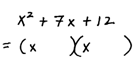Step 2: Factor the last term. Choose factors that add or subtract to obtain the middle term.Step 3: Determine the signs by adding or subtracting the product of the inner and outer terms.Step 4: Check by multiplying.Rather than trying all possible combinations of the factors that make up the last term spend some time looking at the factors before starting step two. Look for combinations that will produce the middle term. Here is the thought process in choosing 3 and 4 in step two above: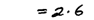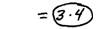"Can I add or subtract 1 and 12 to obtain 7?" – NO
"Can I add or subtract 2 and 6 to obtain 7?" – NO
"Can I add or subtract 3 and 4 to obtain 7?" – YES, because +3 + 4 = +7

Factor the trinomials.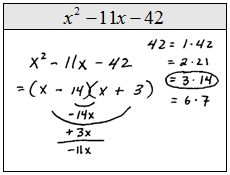This process used for factoring trinomials is sometimes called guess and check or trial and error. The biggest problem occurs when the signs are improperly chosen. With this in mind, you should take care to check your results by multiplying. Also, since multiplication is commutative order does not matter, in other words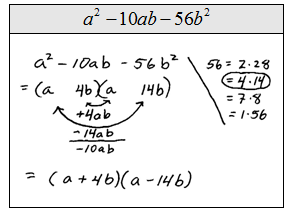If the trinomial has a GCF you should factor that out first.  Also, you should factor in such a way as to ensure a resulting trinomial with a positive leading coefficient.

Factor the trinomials.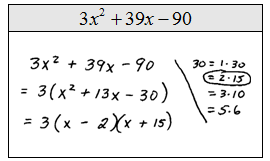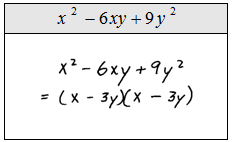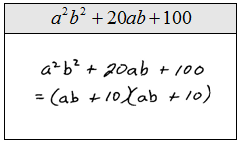Take care to perform the check. Most of the problems that you will encounter factor nicely but be sure to watch out for something like this . The middle term works but the last term does not:because the sign of the last term is incorrect